# Rounding Worksheets

#### How to Round Numbers and Why Do We Do It

Doing math is no walk in the park, and when it comes to rounding off numbers – the simplest concept – you can strengthen your grip on the subject. You round numbers to their closest to their original values to make calculations simpler. When calculation results come in decimals or calculations appear complex, you can round the numbers to their nearest ten. While there are some steps and calculations you have to follow, the nearest ten is the easiest one out of all. Consider the examples below to understand better: 73 → 70 | 124.9 → 120 | 81 → 80 | 134.9 → 130. Here we look at the considerations for rounding number: Consider which the last digit you want to keep. If the digit before the last digit is less than five, leave it the same. (This is most commonly known is called rounding down technique.) When the number before the last digit is more than five, increase the number by 1. (Rounding up!)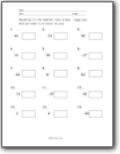###### Tens Place (w/ Negatives)

Round each number to the nearest 10s place.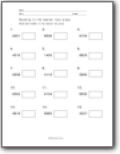###### Tens Place (2-Digit Values)

Round each number to the nearest 10s place.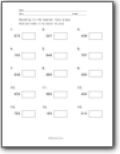###### Tens Place (3-Digit Values)

Round each number to the nearest 10s place.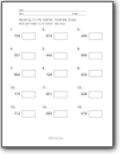###### Hundreds Place (3-Digit Values)

Round each 3-digit numbers to the nearest 100s place.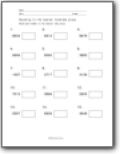###### The Hundreds Place (4-Digit Values)

Round each 4-digit numbers to the nearest 100s place.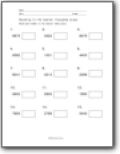###### 4-Digits Nearest Thousands

Round each number to the nearest 1000s place.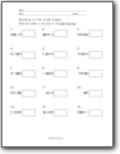###### Mixed Places

Round each number to the place of the underlined digit.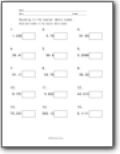###### Decimals to Whole Numbers

Round each number to the nearest whole number.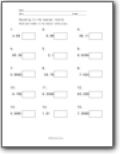###### Decimals (Tenths)

Round each decimal value to the nearest tenths place.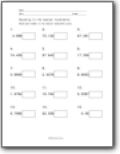###### Decimals (Hundredths)

Round each number to the nearest hundredths place.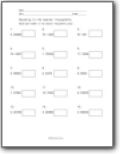###### Decimals (Thousandths)

You know what you need to do at this point.